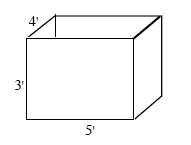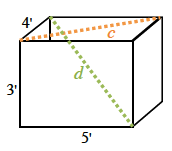### Home > A2C > Chapter 8 > Lesson 8.1.4 > Problem8-71

8-71.

A $5' \times 4' \times 3'$box is made for the purpose of storage. What is the longest pole that can fit inside the box?Find the distance between two opposite corners of the box.

Draw a right triangle on the base of the box.
Find the measure of the hypotenuse.

$4^² + 5^² = c^²$Now draw a right triangle from the bottom corner to the top of the box.
Find the measure of that hypotenuse.

$c^² + 3^² = d^²$

$7.07'$